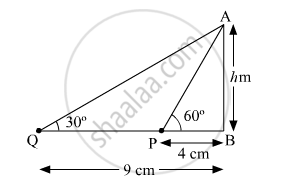The Angles of Elevation of the Top of a Tower from Two Points at a Distance of 4 M and 9 M from the Base of the Tower and in the Same Straight Line with It Are 60° and 30° Respectively. Find the Height of the Tower. - Mathematics

The angles of elevation of the top of a tower from two points at a distance of 4 m and 9 m from the base of the tower and in the same straight line with it are 60° and 30° respectively. Find the height of the tower.

Solution

Let the height of the tower be h m.In ABP,

tan60^@ = h/4

=>sqrt3=h/4

h=1.73×4=6.92 cm

In ABQ

tan30^@=h/9

=>1/sqrt3=h/9

=>h=9/sqrt3cm= 5.20 cm

Using the given data, we are getting two different values of h, which is not possible.

Concept: Heights and Distances
Is there an error in this question or solution?